A versatile cross-platform mind mapping tool.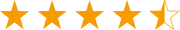Get from App StoreA versatile cross-platform mind mapping tool.Get from App Store
edraw-max-horizontal
Product Tour
Guide
What's New
EdrawMax Online
TRY IT FREE

# Explain Algorithm and Flowchart with Examples

> Flowchart Examples > Explain Algorithm and Flowchart with Examples
Posted by Allison Lynch | 08/17/2020
The algorithm and flowchart are two types of tools to explain the process of a program. In this page, we discuss the differences between an algorithm and a flowchart and how to create a flowchart to illustrate the algorithm visually.

Algorithms and flowcharts are two different tools used for creating new programs, especially in computer programming. An algorithm is a step-by-step analysis of the process, while a flowchart explains the steps of a program in a graphical way.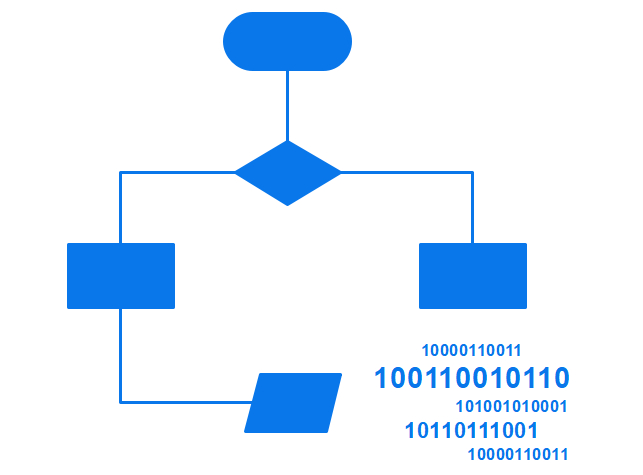### Definition of Algorithm

To write a logical step-by-step method to solve the problem is called the algorithm; in other words, an algorithm is a procedure for solving problems. In order to solve a mathematical or computer problem, this is the first step in the process. An algorithm includes calculations, reasoning, and data processing. Algorithms can be presented by natural languages, pseudocode, and flowcharts, etc.

### Definition of Flowchart

A flowchart is the graphical or pictorial representation of an algorithm with the help of different symbols, shapes, and arrows to demonstrate a process or a program. With algorithms, we can easily understand a program. The main purpose of using a flowchart is to analyze different methods. Several standard symbols are applied in a flowchart:

 Terminal Box - Start / End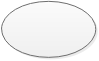Input / OutputProcess / InstructionDecision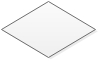Connector / Arrow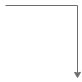The symbols above represent different parts of a flowchart. The process in a flowchart can be expressed through boxes and arrows with different sizes and colors. In a flowchart, we can easily highlight certain elements and the relationships between each part.

### Difference between Algorithm and Flowchart

If you compare a flowchart to a movie, then an algorithm is the story of that movie. In other words, an algorithm is the core of a flowchart. Actually, in the field of computer programming, there are many differences between algorithm and flowchart regarding various aspects, such as the accuracy, the way they display, and the way people feel about them. Below is a table illustrating the differences between them in detail.

Algorithm Flowchart
It is a procedure for solving problems. It is a graphic representation of a process.
The process is shown in step-by-step instruction. The process is shown in block-by-block information diagram.
It is complex and difficult to understand. It is intuitive and easy to understand.
It is convenient to debug errors. It is hard to debug errors.
The solution is showcased in natural language. The solution is showcased in pictorial format.
It is somewhat easier to solve complex problem. It is hard to solve complex problem.
It costs more time to create an algorithm. It costs less time to create a flowchart.

### Types of Algorithm

It is not surprising that algorithms are widely used in computer programming. However, it can be applied to solving mathematical problems and even in everyday life. Here comes a question: how many types of algorithms? According to Dr. Christoph Koutschan, a computer scientist working at the Research Institute for Symbolic Computation (RISC) in Austria, he has surveyed voting for the important types of algorithms. As a result, he has listed 32 crucial algorithms in computer science. Despite the complexity of algorithms, we can generally divide algorithms into six fundamental types based on their function.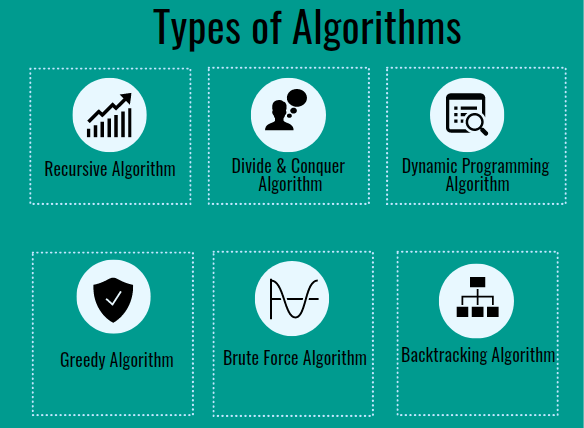Source image: www.educba.com

1. Recursive Algorithm

It refers to a way to solve problems by repeatedly breaking down the problem into sub-problems of the same kind. The classic example of using a recursive algorithm to solve problems is the Tower of Hanoi.

2. Divide and Conquer Algorithm

Traditionally, the divide and conquer algorithm consists of two parts: 1. breaking down a problem into some smaller independent sub-problems of the same type; 2. finding the final solution of the original issues after solving these more minor problems separately.

The key points of the divide and conquer algorithm are:

• If you can find the repeated sub-problems and the loop substructure of the original problem, you may quickly turn the original problem into a small, simple issue.
• Try to break down the whole solution into various steps (different steps need different solutions) to make the process easier.
• Are sub-problems easy to solve? If not, the original problem may cost lots of time.

3. Dynamic Programming Algorithm

Developed by Richard Bellman in the 1950s, the dynamic programming algorithm is generally used for optimization problems. In this type of algorithm, past results are collected for future use. Like the divide and conquer algorithm, a dynamic programming algorithm simplifies a complex problem by breaking it down into some simple sub-problems. However, the most significant difference between them is that the latter requires overlapping sub-problems, while the former doesn’t need to.

4. Greedy Algorithm

This is another way of solving optimization problems – greedy algorithm. It refers to always finding the best solution in every step instead of considering the overall optimality. That is to say, what he has done is just at a local optimum. Due to the limitations of the greedy algorithm, it has to be noted that the key to choosing a greedy algorithm is whether to consider any consequences in the future.

5. Brute Force Algorithm

The brute force algorithm is a simple and straightforward solution to the problem, generally based on the description of the problem and the definition of the concept involved. You can also use "just do it!" to describe the strategy of brute force. In short, a brute force algorithm is considered as one of the simplest algorithms, which iterates all possibilities and ends up with a satisfactory solution.

6. Backtracking Algorithm

Based on a depth-first recursive search, the backtracking algorithm focusing on finding the solution to the problem during the enumeration-like searching process. When it cannot satisfy the condition, it will return “backtracking” and tries another path. It is suitable for solving large and complicated problems, which gains the reputation of the “general solution method.” One of the most famous backtracking algorithm example it the eight queens puzzle.

### How to Use Flowcharts to Represent Algorithms

Now that we have the definitions of algorithm and flowchart, how do we use a flowchart to represent an algorithm? To create an algorithm, we need to download software.

## EdrawMax: a swiss knife for all your diagramming need

• Effortlessly create over 280 types of diagrams.
• Provide various templates & symbols to match your needs.
• Drag and drop interface and easy to use.
• Customize every detail by using smart and dynamic toolkits.
• Compatible with a variety of file formats, such as MS Office, Visio, PDF, etc.
• Feel free to export, print, and share your diagrams.

Algorithms are mainly used for mathematical and computer programs, whilst flowcharts can be used to describe all sorts of processes: business, educational, personal, and algorithms. So flowcharts are often used as a program planning tool to organize the program's step-by-step process visually. Here are some examples:

#### Example 1: Print 1 to 20:

Algorithm:

Step 1: Initialize X as 0,

Step 2: Increment X by 1,

Step 3: Print X,

Step 4: If X is less than 20 then go back to step 2.

Flowchart: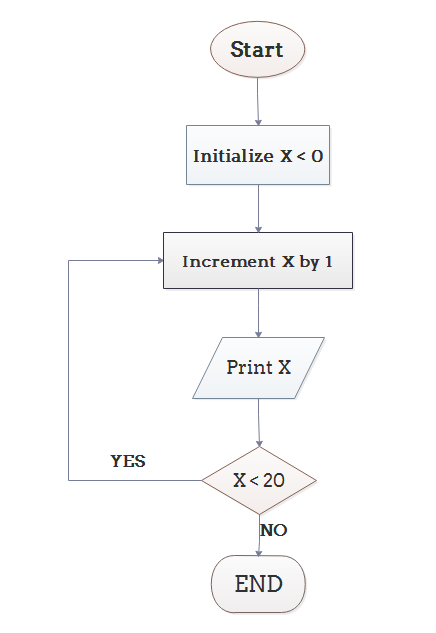#### Example 2: Convert Temperature from Fahrenheit (℉) to Celsius (℃)

Algorithm:

Step 1: Read temperature in Fahrenheit,

Step 2: Calculate temperature with formula C=5/9*(F-32),

Step 3: Print C,

Flowchart: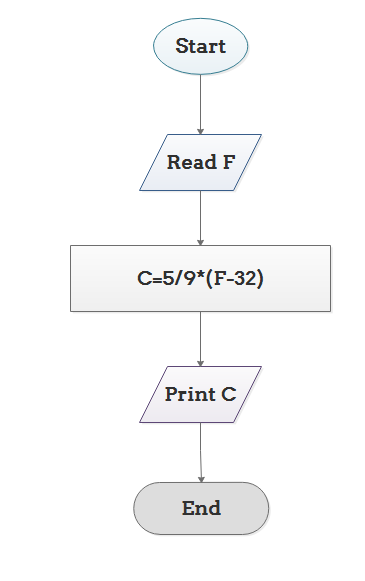#### Example 3: Determine Whether A Student Passed the Exam or Not:

Algorithm:

• Step 1: Input grades of 4 courses M1, M2, M3 and M4,
• Step 3: If the average grade is less than 60, print "FAIL", else print "PASS".

Flowchart: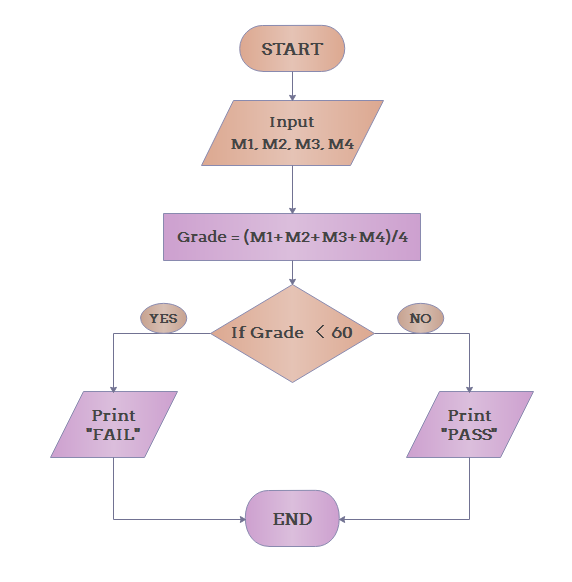### Conclusion

From the above, we can come to the conclusion that a flowchart is a pictorial representation of an algorithm, an algorithm can be expressed and analyzed through a flowchart.

An algorithm shows you every step of reaching the final solution, while a flowchart shows you how to carry out the process by connecting each step. An algorithm uses mainly words to describe the steps while a flowchart uses the help of symbols, shapes and arrows to make the process more logical.

## Get Started! You Will Love This Easy-To-Use Diagram Software

EdrawMax is an advanced all-in-one diagramming tool for creating professional flowcharts, org charts, mind maps, network diagrams, UML diagrams, floor plans, electrical diagrams, science illustrations, and more. Just try it, you will love it!